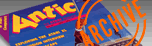`ANTIC VOL. 2, NO. 7 / OCTOBER 1983`

# LINE ZERO

## A little file protection scheme

by BOB FENIGER

This one line program will prevent your programs from being listed once they have been run. Although not a serious file guard, LINE ZERO will confound anyone without knowledge of the program unless they happen to use the SYSTEM RESET key. This last puts the Entertainment label on the program. Just follow the nine step-by-step instructions. Step 3 contains the entire LINEZERO program.

1. LOAD or ENTER a program from disk or cassette. In this example, we will call your program NOTES.

2. LIST the first few lines of NOTES. If there is a line 0, renumber it to a higher number. Otherwise, make no changes.

3. Type in line 0, exactly as follows

O POKE 694,9:POKE 755,4:POKE 756,0:STOP

4. This line is now included in the program. Save this new program as "NOTES.II" if you have a disk drive, otherwise CSAVE to a diifferent tape.

5. When the new program is saved, switch the ATARI off, and then on again.

6. Type RUN "D:NOTES.II" or rewind the second tape and type RUN"C:" An unusual pattern should now be displayed. Don't be concerned - this is part of the protection.

7. Press [BREAK] two or three times. You won't see the display change much, but that's expected. Now type this:

YFBL>%lt;?%;;= press [RETURN].

The screen should clear at this point. If not, press [BREAK] two more times and try again. When you clear the screen, you won't need a mirror to read it if you enter these nine characters:

YFBL>%lt;%8 press [RETURN]

8. Now key in 9. This is the program code for 0 and thus will delete line 0. You should now be able to LIST, RUN, DOS, etc. Although line 0 is no longer in the version of NOTES.II that resides in the computer, it is still in the SAVEd version. If you wish to edit, remember to replace line 0 before you SAVE again.

9. To exit the code, type:

YFBL?0=%9 press [RETURN]

This will return the regular ATARI character set.

By skipping the ninth step you can LIST, RUN, DOS, etc. by using this code table. It is possible to even write new lines or edit existing lines by using the code table. Try E@Z] for LIST, [+G for RUN, and MFZ for DOS. If you access DOS the code set will still be in effect. [SYSTEM RESET] will return you to BASIC as well as restoring the normal character set. [RESET] will solve most problems if things get a bit clogged up. Any program may be similarly protected by adding LINE ZERO and repeating the above steps. Here is part of the character set code that results when a 9 is POKEd into memory location 694.

A=H, B=K, C=J, D=M, E=L, F=O, G=N, H=A, I=@, J=C, K=B, L=E, M=D, N=G, O=F, P=Y, Q=X, R=[, S=Z, T=], U=\, V=_, W=A, X=Q, Y=P, Z=S, 0=9, 1=8, 2=; 3=:, 4==, 5=<, 6=?, 7=>, 8=1, 9=0, ,=%, "=+, (SQUARE ROOT SIGN)=', 0=W, [=R, ]=T, \=U, ==4, _=V, :=3,;=2, ?=6, %lt;=5, >=7, !=( (=!, #=*, *=#, \$=-, &=/, /=&, space bar=), )=blank space